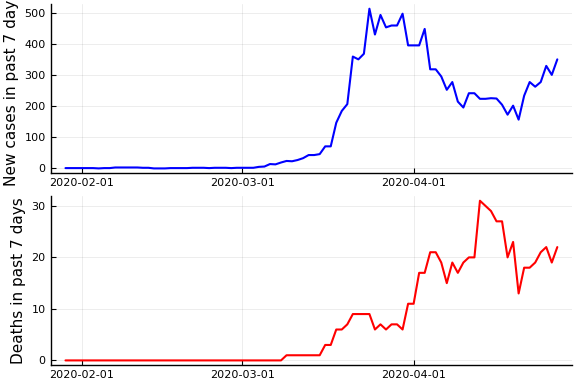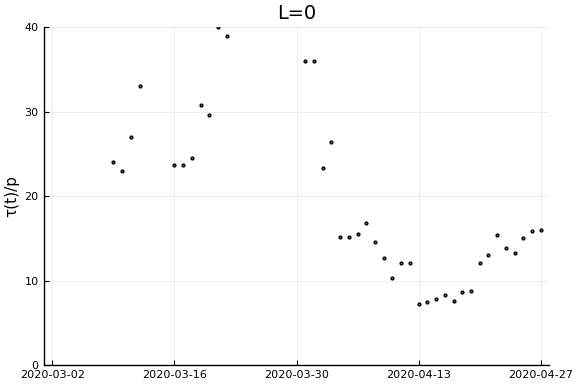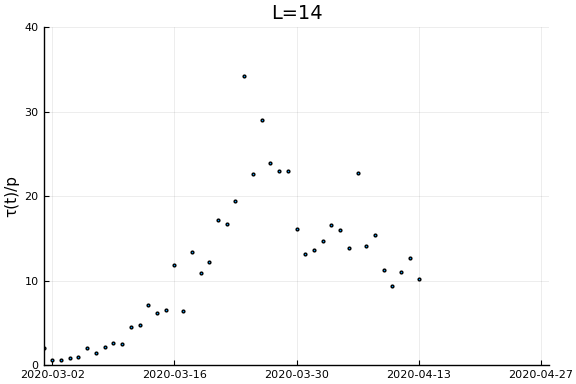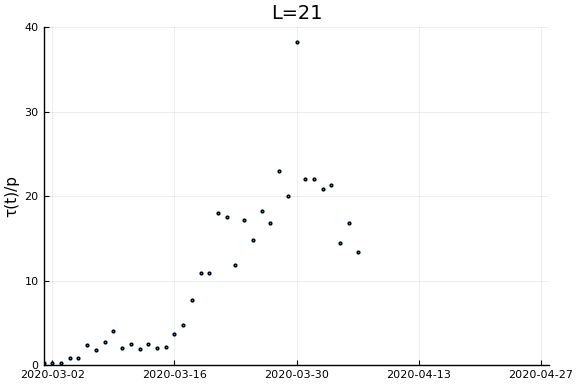This note describes how changes in the relationship between new confirmed cases and deaths can be used to infer changes in testing.

To illustrate the idea, begin by considering a simple SIR model with the addition that infections get detected at rate $\tau(t)$.1

where $S$ is susceptible, $I$ is infections, $C$ is cumulative confirmed cases, and $D$ are deaths. Then we immediately have that

Assuming death rates are constant, then any departure from a linear relationship between $\dot{C}$ and $\dot{D}$ is due to changes in testing.

# Time delays¶

The basic SIR model assume the hazard rate of death does not dependent on infection duration. This is a poor assumption, and, moreover, affects the relationship between $\dot{C}$ and $\dot{D}$. To illusture, we will assume a simple form of duration dependence – infections last $\ell$ days. After $\ell$ days a person dies with probability $p_D$. We will also change the process generating confirmed cases to new infection on day $t$ are detected with probability $\tau(t)$. The system now evolves as

Rearranging we have

Again, assuming the probability of death is constant, any departure from a linear relationship between $\dot{C}(t)$ and $\dot{D}(t-\ell)$ is due to changes in testing.

# General Relationship¶

Other modifications to the model, such as an additional incubation period or random infection durations will lead to slightly different implications about the relationship betwen $\tau$, $\dot{C}$, and $\dot{D}$. However, it will always be of the form (now I’m treating time as discrete, replace the sum with an integral if you prefer continuous time):

Where $\tilde{p}_\ell$ are from inverting the relationship

where $\underline{\ell}$ is the smallest $\ell$ such that $P(\text{die time } t) | \text{infected time } t-\ell) > 0$, and assuming that death probabilities are do not depend on time of infection.

Then substituting into

gives

# Data¶

using CovidSEIR, Plots, Dates
Plots.pyplot()
df = CovidSEIR.covidjhudata()
sdf=filter(x->(!ismissing.(x.Province) .& (x.Province .== "British Columbia")), df)
Δ=7
date = sdf.Date[(Δ+1):end]
newcases = sdf.confirmed[(Δ+1):end] .- sdf.confirmed[1:(end-Δ)]
deaths = sdf.deaths[(Δ+1):end] .- sdf.deaths[1:(end-Δ)]

plot( plot(date, newcases, ylabel="New cases in past $Δ days", color="blue", linewidth=1.5, legend=:none), plot(date, deaths, ylabel="Deaths in past$Δ days", color="red", linewidth=1.5, legend=:none), layout=(2,1))Now if we assume the time from testing to death is $L$ days, then $\tau(t)/p_D = \frac{\dot{C}(t)}{\dot{D}(t+L)}$. Let’s plots this for a variety of $L$.

xlim = Dates.value.((Date("2020-03-01"), Dates.today()))
ylim = (0, 40)
ylab = "τ(t)/p"
for L in [0, 3, 7, 14, 21]
display(scatter(date[1:(end-L)], newcases[1:(end-L)]./deaths[(L+1):end], ylim=ylim, xlim=xlim,
legend=:none, ylabel=ylab, title="L=$L", markersize=2, reuse=false)) end1. Whether you treat time as continuous and read$\dot{S} = dS/dt$or treat time as discrete and read$\dot{S} = S(t) - S(t-1)\$ makes no difference for anything in this note.